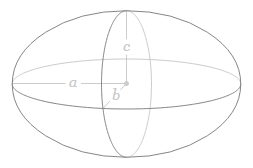## Volume of a Ellipsoid Calculator

 Unit: Inches Metres Centimetres Millimetres Yards Feet Radius a: Radius b: Radius c: Results: Volume Of Ellipsoid:

An ellipsoid is a closed quadric surface that is a three-dimensional analogue of an ellipse.### Volume of Ellipsoid formula V=4/3(πabc)

For example, when a = 1, b = 2, c = 3, then V ≈ 25.1327412287184.

Thinkcalculator.com provides you helpful and handy calculator resources.